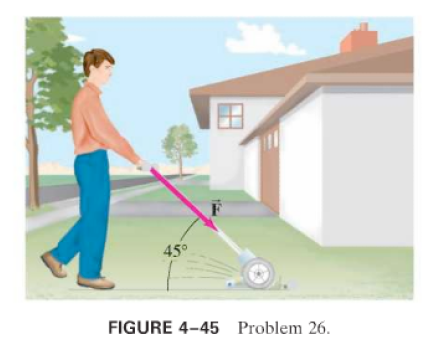×
Get Full Access to Physics: Principles With Applications - 6 Edition - Chapter 4 - Problem 26p
Get Full Access to Physics: Principles With Applications - 6 Edition - Chapter 4 - Problem 26p

×

(II) A person pushes a 14.0-kg lawn mower at constantISBN: 9780130606204 3

Solution for problem 26P Chapter 4

Physics: Principles with Applications | 6th Edition

• Textbook Solutions
• 2901 Step-by-step solutions solved by professors and subject experts
• Get 24/7 help from StudySoup virtual teaching assistantsPhysics: Principles with Applications | 6th Edition

4 5 1 395 Reviews
30
3
Problem 26P

(II) A person pushes alawn mower at constant speed with a force ofdirected along the handle, which is at an angle ofto the horizontal (Fig.. (a) Draw the free-body diagram showing all forces acting on the mower. Calculate (b) the horizontal friction force on the mower, then (c) the normal force exerted vertically upward on the mower by the ground. () What force must the person exert on the lawn mower to accelerate it from rest toinseconds, assuming the same friction force?Step-by-Step Solution:

Solution 26P: We have to calculate the forces exerted on lawn mower and the forces exerted by the lawn mower under the given situation. Step 1 of 5 Concept: 1) Newton’s third law: To every action there is an equal and opposite reaction. 2) Newton’s second law: The force F acts on mass m produces an acceleration a in the object. Mathematically, F=ma. 3) Frictional force also acts on the surface of the bodies in contact whenever their is relative motion between between the two bodies. 4) Free body diagram of a body gives the diagrammatical representation of all the forces acting on the body in terms of magnitude and diagram, under the given situation.

Step 2 of 5

Step 3 of 5

ISBN: 9780130606204

Unlock Textbook Solution